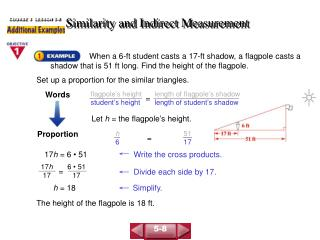DownloadDownload PresentationSimilarity and Indirect Measurement

# Similarity and Indirect Measurement

Download Presentation## Similarity and Indirect Measurement

- - - - - - - - - - - - - - - - - - - - - - - - - - - E N D - - - - - - - - - - - - - - - - - - - - - - - - - - -
##### Presentation Transcript

1. flagpole’s height student’s height length of flagpole’s shadow length of student’s shadow Words Let h = the flagpole’s height. = h 6 51 17 Proportion = 17h = 6 • 51 Write the cross products. 17h 17 6 • 51 17 = Divide each side by 17. h = 18 Simplify. COURSE 3 LESSON 5-8 Similarity and Indirect Measurement When a 6-ft student casts a 17-ft shadow, a flagpole casts a shadow that is 51 ft long. Find the height of the flagpole. Set up a proportion for the similar triangles. The height of the flagpole is 18 ft. 5-8

2. Use similar triangles to set up a proportion involving the lengths of corresponding sides. ED corresponds to AB. CD corresponds to CB. ED AB CD CB = d 416 141 312 Substitute. = Write the cross products. 312 • d = 416 • 141 COURSE 3 LESSON 5-8 Similarity and Indirect Measurement In the figure below, ABC ~ EDC. Find d. 5-8

3. 312d 58,656 312 312 Divide each side by 312. = Use a calculator. 58,656 312 188 COURSE 3 LESSON 5-8 Similarity and Indirect Measurement (continued) Simplify. 312d = 58,656 The length of d is 188 m. 5-8

4. COURSE 3 LESSON 5-8 Similarity and Indirect Measurement 1. A 5-ft tall student casts a 12-ft shadow. A tree casts a 27-ft shadow. How tall is the tree? 2. A 6-ft man casts a 9-ft shadow. A sculpture casts a 45-ft shadow. How tall is the sculpture? 11.25 ft tall 30 ft 5-8